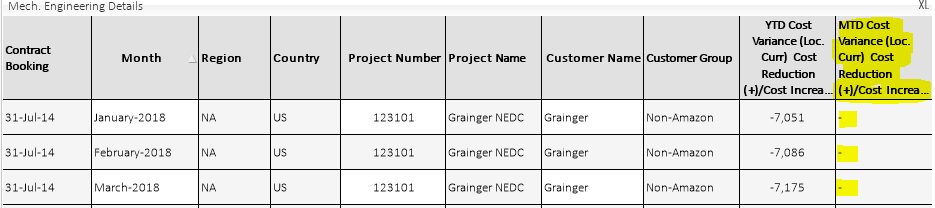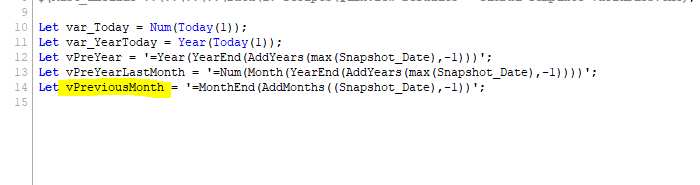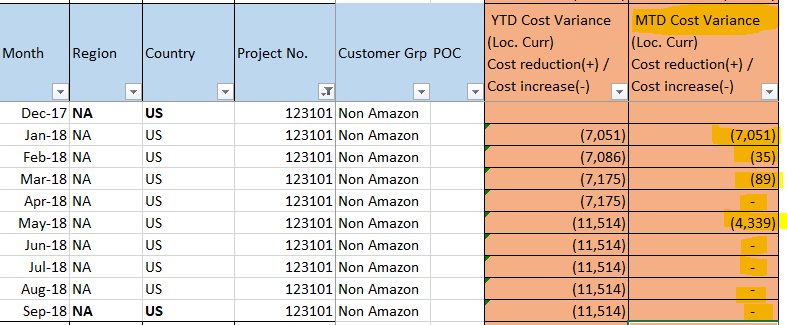# New to QlikView

Discussion board where members can get started with QlikView.

Highlighted
Contributor III

## Set Analysis for Current month Value minus Previous month Value

Hello,

I have the table as shown below;I am trying to calculate the MTD Variance which is (Current month YTD) - (Previous month YTD) with the expression as;

Sum(YTD)

-

Sum({\$< Snapshot_Date = {\$(vPreviousMonth)}>}YTD)

where PreviousMonth is a variable defined as

The above expression returns null values, what wrong am I doing here? any help is appreciated

thanks

Tags (4)
1 Solution

Accepted SolutionsMVP

## Re: Set Analysis for Current month Value minus Previous month Value

Check attached.... you don't need set analysis here... but Inter-Record Functions

15 RepliesMVP

## Re: Set Analysis for Current month Value minus Previous month Value

Can you show the image with this variable vPreviousMonth in text box?

Before develop something, think If placed (The Right information | To the right people | At the Right time | In the Right place | With the Right context)MVP

## Re: Set Analysis for Current month Value minus Previous month Value

May be try this

LET vPreviousMonth = '=Date(Floor(MonthEnd(Snapshot_Date, -1)))';

Contributor III

## Re: Set Analysis for Current month Value minus Previous month Value

This is how I defined the variable.Contributor III

## Re: Set Analysis for Current month Value minus Previous month Value

The values are still blank. I have used your variable and this expression;

Sum(YTD)

-

Sum({\$< Snapshot_Date = {\$(vPreviousMonth)}>}YTD)MVP

## Re: Set Analysis for Current month Value minus Previous month Value

Try this

Sum(YTD) - Sum({\$< Snapshot_Date = {"\$(vPreviousMonth)"}>}YTD)

or

Sum(YTD) - Sum({\$< Snapshot_Date = {"\$(=vPreviousMonth)"}>}YTD)

or

Sum(YTD) - Sum({\$< Snapshot_Date = {"\$(=\$(vPreviousMonth))"}>}YTD)

Contributor III

## Re: Set Analysis for Current month Value minus Previous month Value

I've tried the expressions which are showing as null values. Attached the file for your reference.MVP

## Re: Set Analysis for Current month Value minus Previous month Value

There is something wrong about your expression.... you have Sum( over Sum( without Aggr()

Sum({\$< Snapshot_Date = {"\$(vPreviousMonth)"}>}

(If(Num_Month = '\$(vPreYearLastMonth)' and Year = '\$(vPreYear)', 0,

((If(Num_Month = '\$(vPreYearLastMonth)' and Year = '\$(vPreYear)', 0, Sum({<Cost_Area = {'Mechanical Engineering (labor + travel)'}>}Updated_OEC_Budget)))

-

(If(Num_Month = '\$(vPreYearLastMonth)' and Year = '\$(vPreYear)', 0, Sum({<Cost_Area = {'Mechanical Engineering (labor + travel)'}>}Forecast_Cost_to_Complete))))

-

((Sum(TOTAL <Cost_Area> {<Year = {'\$(vPreYear)'} , Num_Month = {'\$(vPreYearLastMonth)'}, Cost_Code = {'3'}, Snapshot_Date = >}Updated_OEC_Budget))

-

(Sum(TOTAL <Cost_Area> {<Year = {'\$(vPreYear)'} , Num_Month = {'\$(vPreYearLastMonth)'}, Cost_Code = {'3'}, Snapshot_Date = >}Forecast_Cost_to_Complete)))))

What exactly are you trying to do here?

Contributor III

## Re: Set Analysis for Current month Value minus Previous month Value

I have that expression to calculate my YTD.

The MTD should be Current month YTD - Previous month YTD as shown below;Contributor III

## Re: Set Analysis for Current month Value minus Previous month Value

I am testing the MTD to get the previous month YTD, so that I can subtract that with the current month YTD.